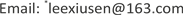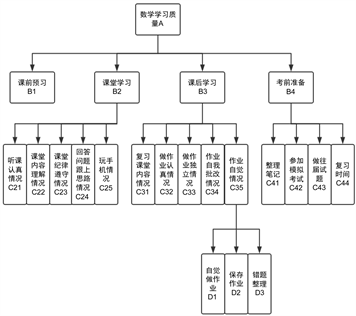﻿ AHP结合多元回归对影响大学生数学学习质量因素的实证分析 An Empirical Study on the Factors Affecting the Quality of College Students’ Mathematics Learning Based on AHP and Regression Analysis

Vol. 08  No. 07 ( 2019 ), Article ID: 31455 , 6 pages
10.12677/ASS.2019.87175

An Empirical Study on the Factors Affecting the Quality of College Students’ Mathematics Learning Based on AHP and Regression Analysis

Qian Zhao, Xiusen Li*, Yue Sun

School of Mathematics and Statistics, Shandong University of Technology, Zibo ShandongReceived: Jul. 3rd, 2019; accepted: Jul. 17th, 2019; published: Jul. 26th, 2019ABSTRACT

Taking the factors that affect the quality of college students’ mathematics learning as indicators, the comprehensive weight is calculated by using the analytic method of tomography, and the weighted sum of all the factors is obtained by using the data of the questionnaire, so as to realize the comprehensive evaluation of the quality of college students’ mathematics learning. SPSS is also used to evaluate the performance and the results of the empirical analysis.

Keywords:College Mathematics, Influencing Factors, Analytic Hierarchy, Regression Analysis

AHP结合多元回归对影响大学生数学学习质量因素的实证分析1. 引言

2. 基于AHP对大学生数学质量的影响因素的分析

2.1. 构建多层次结构模型Figure 1. Hierarchical chart of influencing factors

2.2. 一致性检验并确定各影响因素的全局权重

$W=\left[\begin{array}{c}0.078343\\ 0.441436\\ 0.166847\\ 0.313374\end{array}\right]$$\begin{array}{l}{\lambda }_{\mathrm{max}}=4.0079\\ CI=0.0026\\ RI=0.90\\ CR=0.0029\end{array}$ $W=\left[\begin{array}{c}0.285714\\ 0.285714\\ 0.071429\\ 0.214286\\ 0.142857\end{array}\right]$$\begin{array}{l}{\lambda }_{\text{max}}=5.0\\ CI=0\\ RI=1.12\\ CR=0\end{array}$

$W=\left[\begin{array}{c}0.277778\\ 0.222222\\ 0.222222\\ 0.111111\\ 0.166667\end{array}\right]$$\begin{array}{l}{\lambda }_{\text{max}}=5.0\\ CI=0\\ RI=1.12\\ CR=0\end{array}$ $W=\left[\begin{array}{c}0.298468\\ 0.203475\\ 0.171101\\ 0.326955\end{array}\right]$$\begin{array}{l}{\lambda }_{\mathrm{max}}=4.228\\ CI=0.0759\\ RI=0.9\\ CR=0.0843\end{array}$

$\begin{array}{l}{\lambda }_{\mathrm{max}}=3.0004\\ CI=0.0002\\ RI=0.58\\ CR=0.0003\end{array}$

C层次总排序的一致性检验：

$CI=\underset{2}{\overset{4}{\sum }}{B}_{i}CI=0.441436×0+166847×0+0.313374×0.0759=0.023785$

$RI=\underset{2}{\overset{4}{\sum }}{B}_{i}RI=0.441436×1.12+0.166847×1.12+0.313374×0.9=0.963314$

$CR=\frac{CI}{RI}=0.0247$

D层次总排序的一致性检验：

$CR=\frac{CI}{RI}=0.0003$

2.3. 各影响因素权重结果分析

3. AHP结合多元回归对影响大学生数学学习质量因素的实证分析

$y=61.551+0.197{x}_{1}+0.215{x}_{2}-0.136{x}_{3}-2.067{x}_{4}$

${x}_{1}$ ：复习课堂内容情况； ${x}_{2}$ ：回答问题跟上思路情况；

${x}_{3}$ ：课前预习情况； ${x}_{4}$ ：考前学习时间。

$y=64.421+0.2{x}_{1}-2.266{x}_{2}-0.112{x}_{3}+0.162{x}_{4}$

${x}_{1}$ ：课堂内容当时理解程度； ${x}_{2}$ ：学习时间；

${x}_{3}$ ：课前预习情况； ${x}_{4}$ ：回答问题跟上思路。

4. 研究建议

An Empirical Study on the Factors Affecting the Quality of College Students’ Mathematics Learning Based on AHP and Regression Analysis[J]. 社会科学前沿, 2019, 08(07): 1275-1280. https://doi.org/10.12677/ASS.2019.87175

1. 1. 彭乃霞, 廖爽, 陈亚萍. 非数学专业大学生数学焦虑成因分析及对策研究[J]. 数学教育学报, 2011, 20(3): 47-50.

2. 2. 陈玉文. 影响大学生数学学习的非智力因素的研究[D]: [硕士学位论文]. 南京: 南京师范大学, 2006.

3. 3. 李红霞. 高等数学自主学习的问题与对策研究[D]: [硕士学位论文]. 重庆: 西南大学, 2009.

4. 4. Weiss, I. (1987) Report of the 1985-86 National Survey of Science and Mathematics Education. Mathematics Education Research.

5. NOTES

*通讯作者。